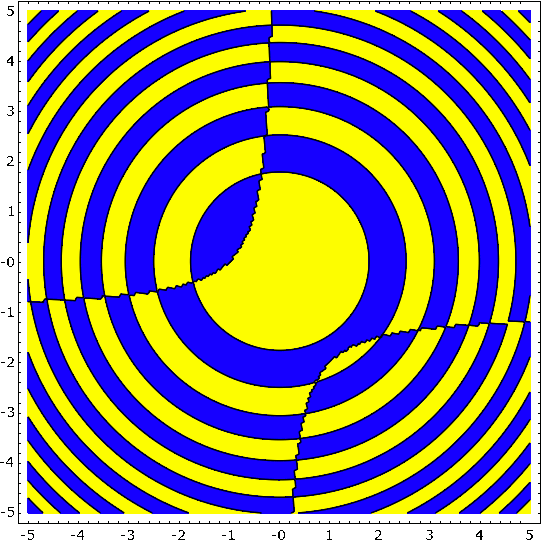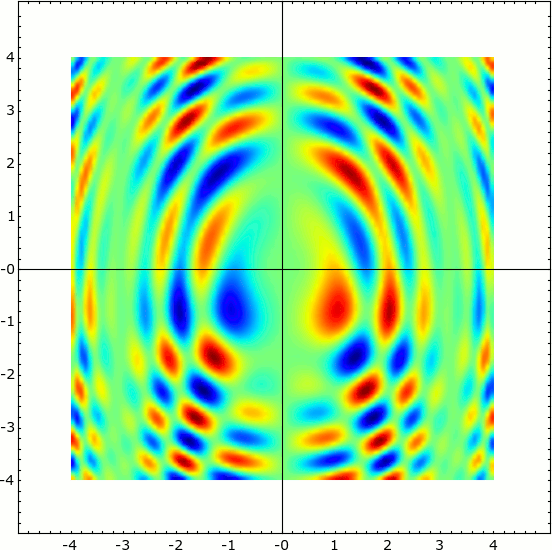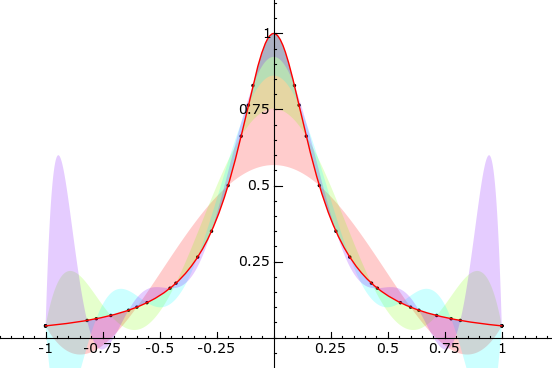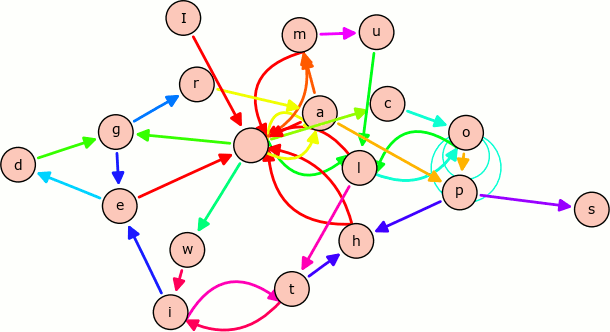# SageMath Plotting and Graphics

SageMath has a wide range of capabilities for visualizing mathematics. It can produce 2-D as well as 3-D graphics, and even animated plots. The wiki contains some examples of images that can be drawn using SageMath.

## Interact

Interact provides an interactive tool to dynamically visualize the impact of parameters on calculations. This is done in a very general way using the basic functionality of Python. Therefore nearly every possible dependency could be shown. The following animation shows a slider on top, which can be dragged in the real case - the plot is then updated accordingly. - see the wiki for more examples

## Regions Plot / Contours

A Region Plot draws those regions, where the given equality, inequality or set of them is true.
$$\huge{\frac{sin(x^2 + y^2)}{(1+y+x y)} > 0}$$sage: var('x y')
sage: region_plot(sin(x^2 + y^2)/(1+y+x*y) > 0, (-5,5), (-5,5), ...
incol='#ffff7f', outcol='#7f7fff', bordercol='black', ...
plot_points=300).show(aspect_ratio=1)


## Density Plot

Density Plot of a two dimensional function.
$$\large{sin(x^2 + y^2) * cos(x+y^2) * sin(y)}$$sage: density_plot(sin(x^2 + y^2) * cos(x+y^2) * sin(y), (-4, 4), (-4, 4), ...
cmap='jet', plot_points=100).show(figsize=(6,6), frame=True)


## Filled Plots

A Filled Plot visualizing approximations of $$\large{ f(x) = \frac{ 1 }{ 1 + 25 x^2 } }$$sage: def f(x):
....:    return RDF(1 / (1 + 25 * x^2))
....:
sage: def runge():
....:       g = plot(f, -1, 1, rgbcolor='red', thickness=1)
....:     polynom = []
....:     for i, n in enumerate([6, 8, 10, 12]):
....:       data = [(x, f(x)) for x in xsrange(-1, 1, 2 / (n - 1), ...
include_endpoint=True)]
....:       polynom.append(maxima.lagrange(data).sage())
....:       g += list_plot(data, rgbcolor='black', pointsize=5)
....:     g += plot(polynom, -1, 1, fill=f, fillalpha=0.2, thickness=0)
....:     return g
....:
sage: runge().show(ymin=0, ymax=1, figsize=(6,4))


## Multiedge Graph

A Multiedge Graph visualizing all pairs of characters in the sentence "I am a cool multiedge graph with loops".sage: stnc = 'I am a cool multiedge graph with loops'
sage: g = DiGraph({}, loops=True, multiedges=True)
sage: for a,b in [(stnc[i], stnc[i + 1]) for i in range(len(stnc) - 1)]: